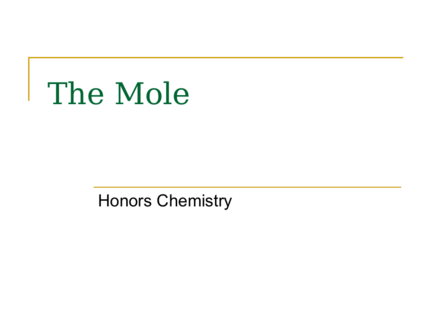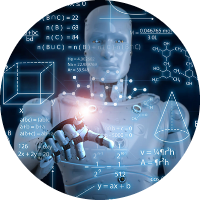# Introduction to the Mole ConceptContributed by:The highlights are:
1. What is a Mole?
2. Units of measurement
3. Mole conversions
1. The Mole
Honors Chemistry
2. Are these moles?
3. What is a mole?
 A unit of measurement
 Since the numbers in chemistry are so small,
we use a “preferred unit of measurement”
(mole) to represent the small number
4. What are the units of
 "that equal number" of atoms arbitrarily chosen.
 the number of atoms in the atomic weight in g of any
element.
 the number of atoms in 16 g of oxygen, in 4 grams
of He, in 32 g of sulfur, etc.
 the number of molecules in the molecular weight, in
grams, of any compound.
 the number of molecules in 18 g of water, in 40 g of
lithium carbide.
 Much later, a mole, that equal number, was found to
be 6.022 x 1023(representative particles).
5.  1 mole (or abbr. mol) = 12.011 g of Carbon
 1 mol of Carbon = 6.02 x 1023 atoms of C
 1 mol of H2O = 6.02 x 1023 molecules of water
 1 mol of NaCl = 6.02 x 1023 units of NaCl
6. Figure 8.1: All these samples of pure elements contain the
same number (a mole) of atoms: 6.022 x 10 23 atoms.
7. Moles in mass
 1 mole can equal the atomic weight of an
element.
 1mol of C = 12.011g of C
 1 mole can equal the atomic weight of a
compound
 1mol of CO = 12.011g of C + 15.99 g of O
= 28.0 g of CO
8. Group Whiteboards
 How many grams are in 1 mol of
 Nitrogen?
 Dinitrogen monoxide?
**How many mols are in 100g sample of N2O?
9. Warm Up #8
 How many grams of Cu are in 1.20 mol of
Cu?
 How many moles are in 70g of Cl?
10. Moles in compounds
Let’s say you have water aka. H2O
How many moles of Hydrogen atoms(ions) are
in water?
 Ask yourself how many individual atoms of
Hydrogen do you see in water?
 Did you say 2???
 You are correct! So how many mols of
Oxygen atoms do you see?
11. Moles in an equation
2 H2 + O2  2H2O
How many moles of Hydrogen molecules are in
the reactants?
*Coefficients serve as the number of moles too!
Therefore, there are 2 mols of Hydrogen gas
12. Units Mole Conversion
Boomerang
13. Mole Conversions
 Mass to mole or vice versa:
 How many moles are in 24 g of Carbon?
 Look at the boomerang:
 What do you want in the end? Moles of C
 Use the conversion factor given:
 1 mole
Atomic mass
24 g of C x 1 mol of C = 2.0 mol of Carbon
12.011 g of C
14. Brain Teaser
 Given 200 g of water, how many molecules of
water do you have?
 How many liters of carbon dioxide are in 1.5 x
10^33 molecules of carbon dioxide?
15. More conversion
 Mole to particles or vice versa
 How many atoms are in 2.00 mols of Carbon?
 Look at the boomerang:
 Where do you begin? (the given: mols)
 What do you need in the end? (atoms: particles)
 What’s the conversion factor?
 6.02 x 1023 Atoms of C
1 Mols of C
2.00 mols of C x 6.02 x 1023 atoms of C = 1.20 x 1024 atoms C
1 mol of C
16. White boards
 How many molecules of oxygen gas are in
3.0 mols of Oxygen gas?
 How many mols of Oxygen gas are in
4.67 x 1024 molecules of Oxygen gas?
17. Final conversion!!!
 Mole to moles of atoms or vice versa
 If you have 2.00 mols of water, how many
moles of hydrogen atoms are there?
 Look at the boomerang:
 Mols  mols of atoms(ions) CF: mols of H+ atoms
mols of water
2.00 mols of H2O x 2 mols of H+ atoms =
1 mol of H2O
= 4.00 mols of H+ atoms
18. Final conversion!!!
 Mole to Volume (gas) or vice versa
 Any gas at STP (standard temperature and
pressure) has a volume of 22.4L per mole
 Look at the cloud chart:
 Mols  volume of gas CF: Moles of gas
22.4L of gas
Ex. How much volume of gas is in 5.00 moles of H2?
5.00 moles H2 x 22.4 L of H2 = 122 L of H2
1 mole of H2
19. Rally table
 Must show work (dimensional analysis)
 How many grams are in 1 mol of Mercury?
 Given a 1.5 mols of carbon dioxide, how
many molecules of carbon dioxide do you
have?
 Given 3.00 mols of carbon tetraflouride, how
many mols of Flourine atoms are there in a
compound?